Examples

Chapter 4 Class 12 Determinants
Serial order wise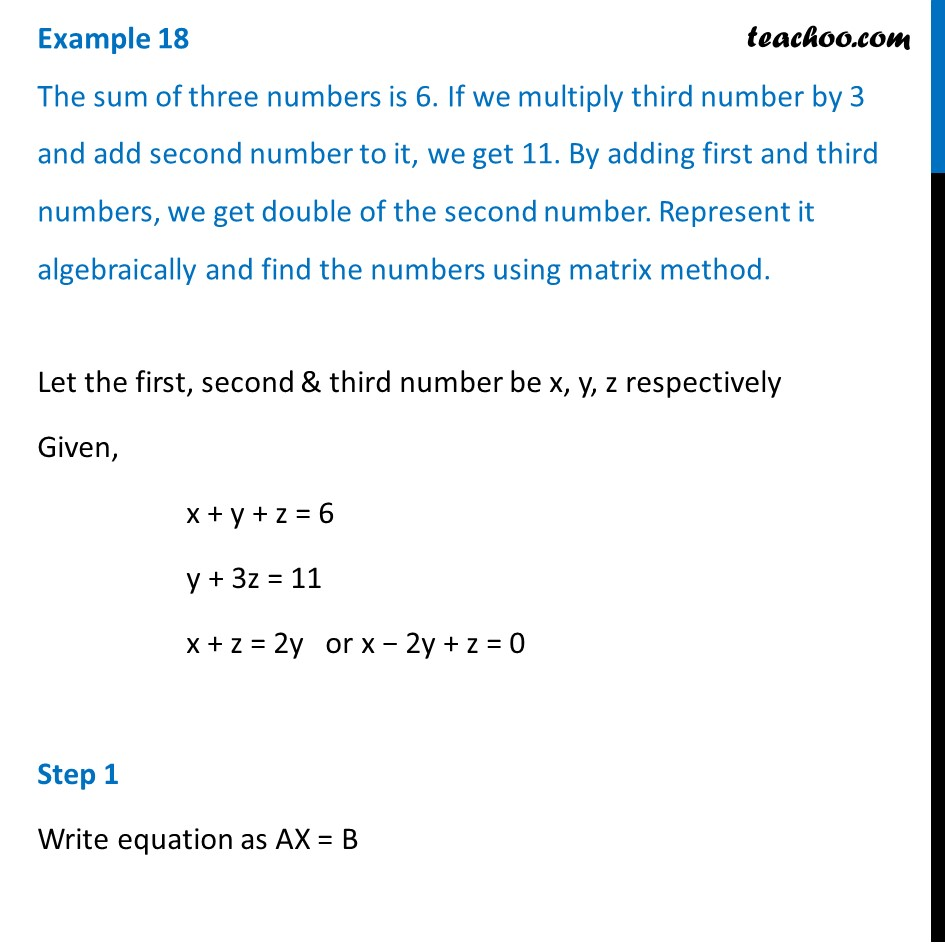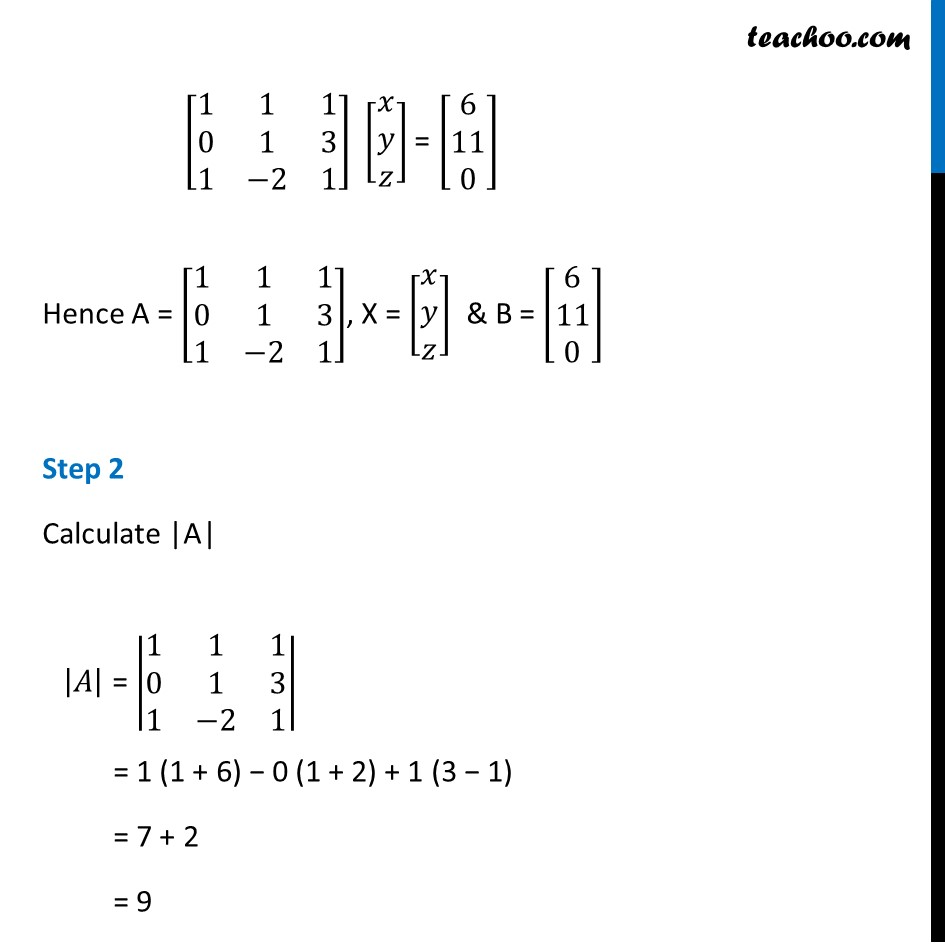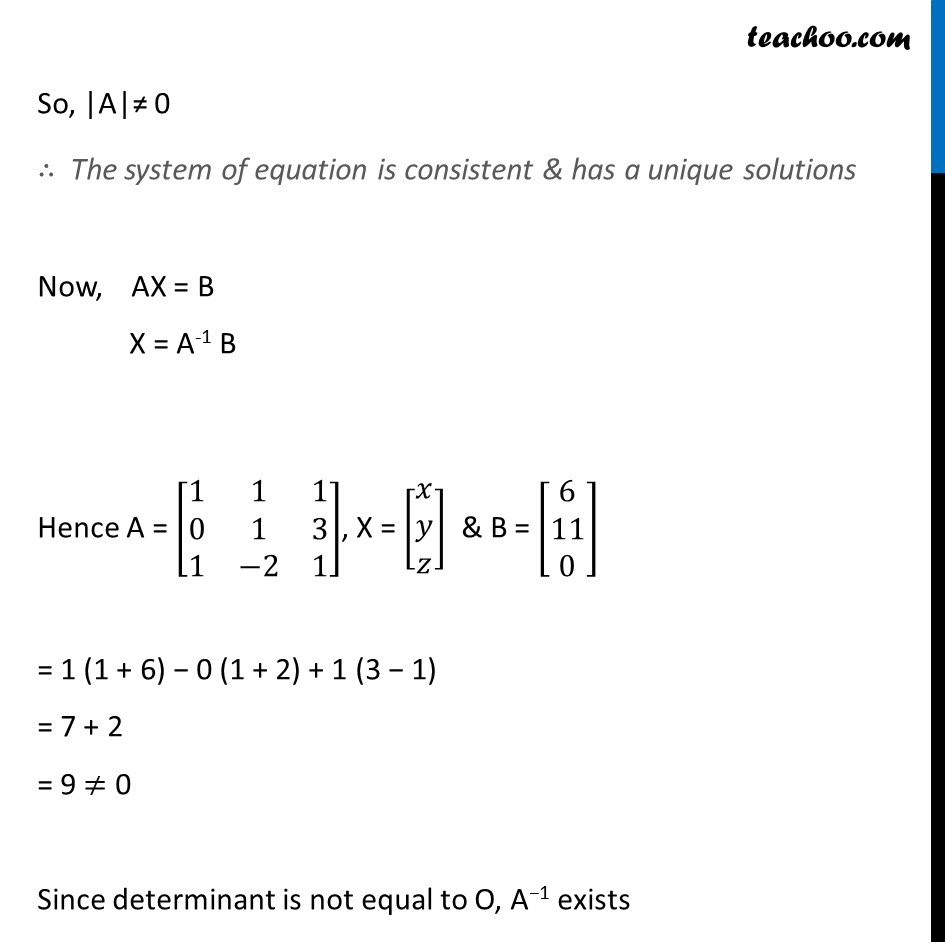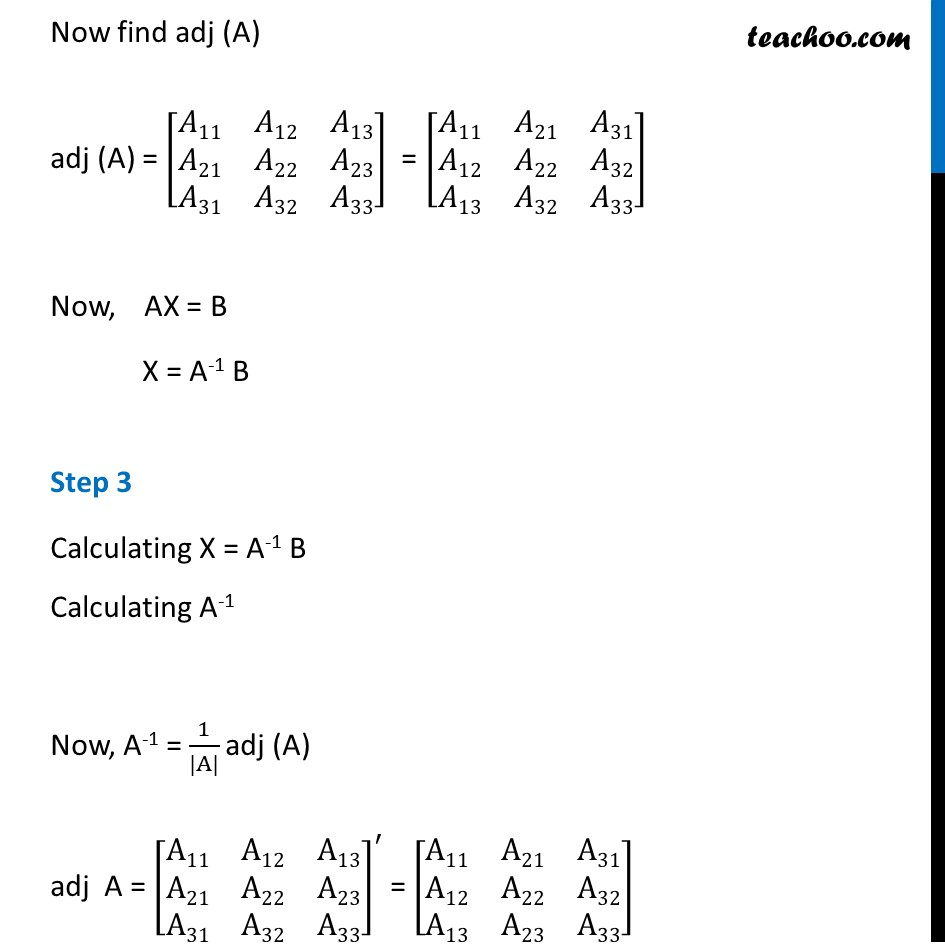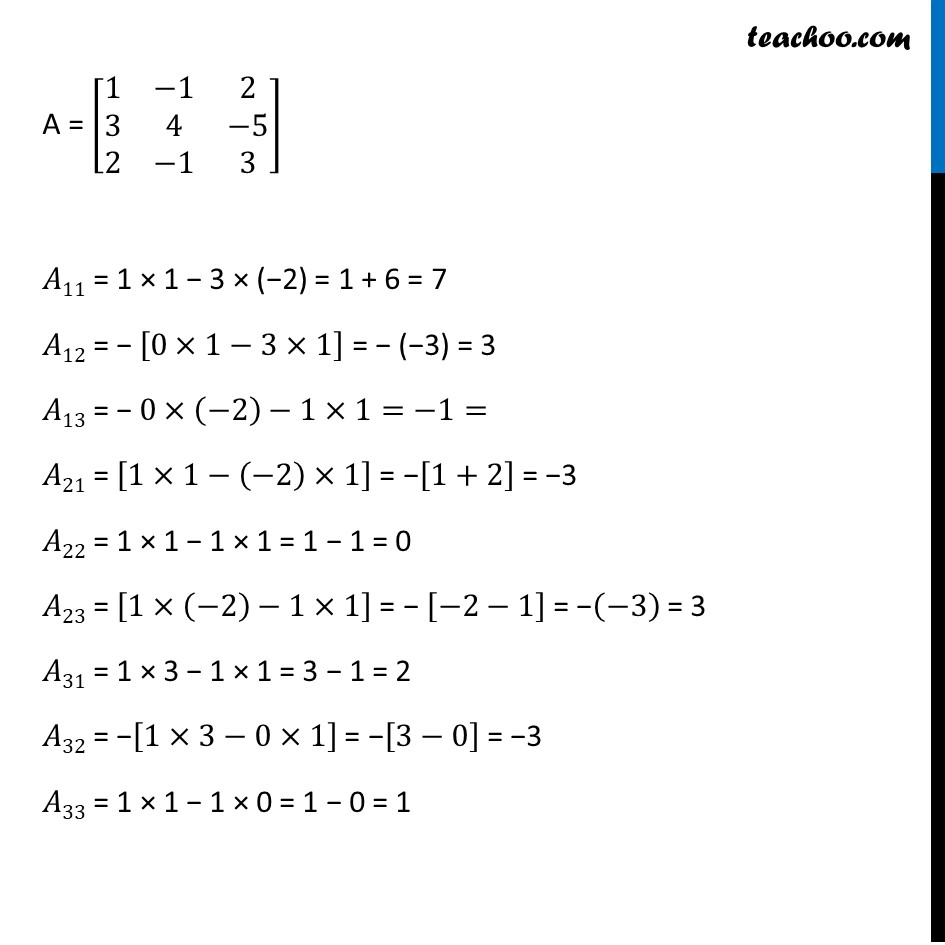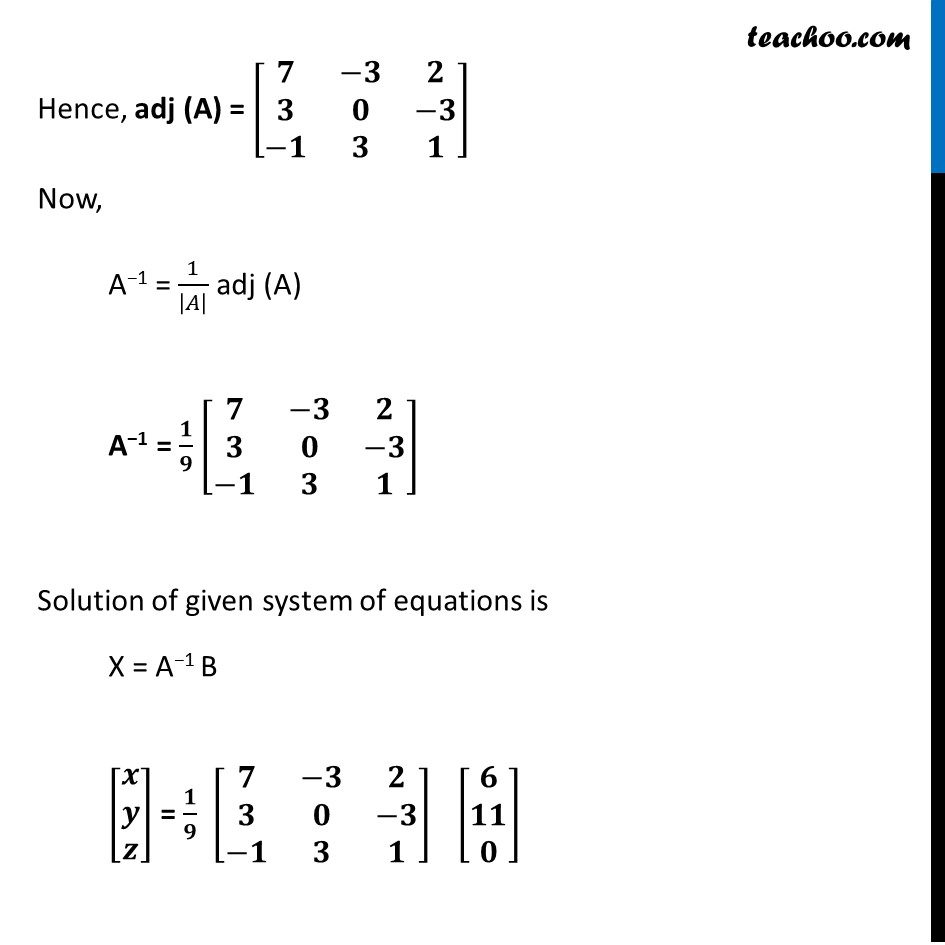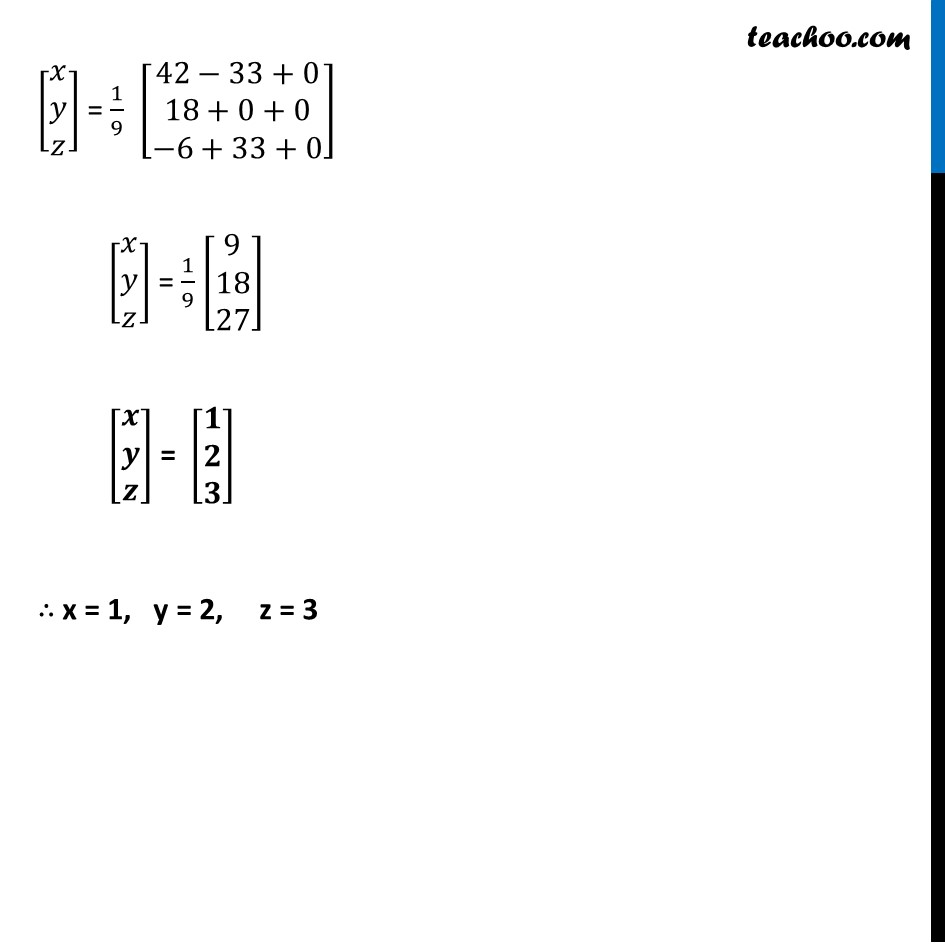Learn in your speed, with individual attention - Teachoo Maths 1-on-1 Class

### Transcript

Example 18 The sum of three numbers is 6. If we multiply third number by 3 and add second number to it, we get 11. By adding first and third numbers, we get double of the second number. Represent it algebraically and find the numbers using matrix method.Let the first, second & third number be x, y, z respectively Given, x + y + z = 6 y + 3z = 11 x + z = 2y or x − 2y + z = 0 Step 1 Write equation as AX = B [■8(1&1&1@0&1&3@1&−2&1)] [■8(𝑥@𝑦@𝑧)] = [■8(6@11@0)] Hence A = [■8(1&1&1@0&1&3@1&−2&1)], X = [■8(𝑥@𝑦@𝑧)] & B = [■8(6@11@0)] Step 2 Calculate |A| |𝐴| = |■8(1&1&1@0&1&3@1&−2&1)| = 1 (1 + 6) − 0 (1 + 2) + 1 (3 − 1) = 7 + 2 = 9 So, |A|≠ 0 ∴ The system of equation is consistent & has a unique solutions Now, AX = B X = A-1 B Hence A = [■8(1&1&1@0&1&3@1&−2&1)], X = [■8(𝑥@𝑦@𝑧)] & B = [■8(6@11@0)] = 1 (1 + 6) − 0 (1 + 2) + 1 (3 − 1) = 7 + 2 = 9 ≠ 0 Since determinant is not equal to O, A−1 exists Now find adj (A) adj (A) = [■8(𝐴11&𝐴12&𝐴13@𝐴21&𝐴22&𝐴23@𝐴31&𝐴32&𝐴33)] = [■8(𝐴11&𝐴21&𝐴31@𝐴12&𝐴22&𝐴32@𝐴13&𝐴32&𝐴33)] Now, AX = B X = A-1 B Step 3 Calculating X = A-1 B Calculating A-1 Now, A-1 = 1/(|A|) adj (A) adj A = [■8(A11&A12&A13@A21&A22&A23@A31&A32&A33)]^′ = [■8(A11&A21&A31@A12&A22&A32@A13&A23&A33)] A = [■8(1&−1&2@3&4&−5@2&−1&3)] 𝐴11 = 1 × 1 − 3 × (−2) = 1 + 6 = 7 𝐴12 = − [0×1−3×1] = − (−3) = 3 𝐴13 = − 0×(−2)−1×1=−1= 𝐴21 = [1×1−(−2)×1] = −[1+2] = −3 𝐴22 = 1 × 1 − 1 × 1 = 1 − 1 = 0 𝐴23 = [1×(−2)−1×1] = − [−2−1] = −(−3) = 3 𝐴31 = 1 × 3 − 1 × 1 = 3 − 1 = 2 𝐴32 = −[1×3−0×1] = −[3−0] = −3 𝐴33 = 1 × 1 − 1 × 0 = 1 − 0 = 1 Hence, adj (A) = [■8(𝟕&−𝟑&𝟐@𝟑&𝟎&−𝟑@−𝟏&𝟑&𝟏)] Now, A−1 = 1/|𝐴| adj (A) A−1 = 𝟏/𝟗 [■8(𝟕&−𝟑&𝟐@𝟑&𝟎&−𝟑@−𝟏&𝟑&𝟏)]" " Solution of given system of equations is X = A−1 B [■8(𝒙@𝒚@𝒛)] = 𝟏/𝟗 [■8(𝟕&−𝟑&𝟐@𝟑&𝟎&−𝟑@−𝟏&𝟑&𝟏)]" " [■8(𝟔@𝟏𝟏@𝟎)] [■8(𝑥@𝑦@𝑧)] = 1/9 [█(42−33+0@18+0+0@−6+33+0)]" " [■8(𝑥@𝑦@𝑧)] = 1/9 [■8(9@18@27)] [■8(𝒙@𝒚@𝒛)] = [■8(𝟏@𝟐@𝟑)] ∴ x = 1, y = 2, z = 3